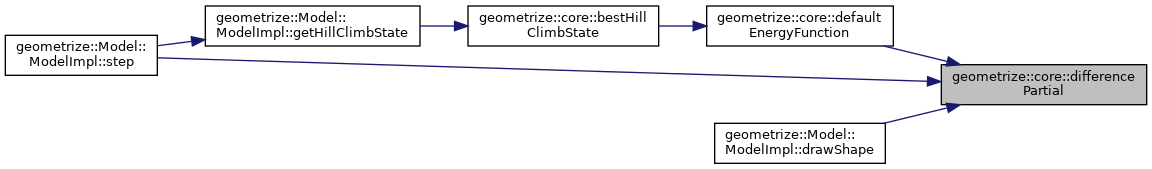Geometrize  1.0 C++ library for geometrizing images into geometric primitives
geometrize::core Namespace Reference

## Typedefs

using EnergyFunction = std::function< double(const std::vector< geometrize::Scanline > &lines, const std::uint32_t alpha, const geometrize::Bitmap &target, const geometrize::Bitmap &current, geometrize::Bitmap &buffer, double score)>
EnergyFunction Type alias for a function that calculates a measure of the improvement adding the scanlines of a shape provides - lower energy is better. More...

## Functions

double defaultEnergyFunction (const std::vector< geometrize::Scanline > &lines, const std::uint32_t alpha, const geometrize::Bitmap &target, const geometrize::Bitmap &current, geometrize::Bitmap &buffer, double score)
defaultEnergyFunction The default/built-in energy function that calculates a measure of the improvement adding the scanlines of a shape provides - lower energy is better. More...

geometrize::rgba computeColor (const geometrize::Bitmap &target, const geometrize::Bitmap &current, const std::vector< geometrize::Scanline > &lines, std::uint8_t alpha)
computeColor Calculates the color of the scanlines. More...

double differenceFull (const geometrize::Bitmap &first, const geometrize::Bitmap &second)
differenceFull Calculates the root-mean-square error between two bitmaps. More...

double differencePartial (const geometrize::Bitmap &target, const geometrize::Bitmap &before, const geometrize::Bitmap &after, double score, const std::vector< Scanline > &lines)
differencePartial Calculates the root-mean-square error between the parts of the two bitmaps within the scanline mask. This is for optimization purposes, it lets us calculate new error values only for parts of the image we know have changed. More...

geometrize::State bestHillClimbState (const std::function< std::shared_ptr< geometrize::Shape >(void)> &shapeCreator, std::uint32_t alpha, std::uint32_t n, std::uint32_t age, const geometrize::Bitmap &target, const geometrize::Bitmap &current, geometrize::Bitmap &buffer, double lastScore, const EnergyFunction &customEnergyFunction=nullptr)
bestHillClimbState Gets the best state using a hill climbing algorithm. More...

## Typedef Documentation

 using geometrize::core::EnergyFunction = typedef std::function& lines, const std::uint32_t alpha, const geometrize::Bitmap& target, const geometrize::Bitmap& current, geometrize::Bitmap& buffer, double score)>

EnergyFunction Type alias for a function that calculates a measure of the improvement adding the scanlines of a shape provides - lower energy is better.

The core functions for Geometrize.

Parameters
 lines The scanlines of the shape. alpha The alpha of the scanlines. target The target bitmap. current The current bitmap. buffer The buffer bitmap. score The score.
Returns
The energy measure.

## Function Documentation

 geometrize::State geometrize::core::bestHillClimbState ( const std::function< std::shared_ptr< geometrize::Shape >(void)> & shapeCreator, std::uint32_t alpha, std::uint32_t n, std::uint32_t age, const geometrize::Bitmap & target, const geometrize::Bitmap & current, geometrize::Bitmap & buffer, double lastScore, const EnergyFunction & customEnergyFunction = `nullptr` )

bestHillClimbState Gets the best state using a hill climbing algorithm.

Parameters
 shapeCreator A function that will create the shapes that will be chosen from. alpha The opacity of the shape. n The number of random states to generate. age The number of hillclimbing steps. target The target bitmap. current The current bitmap. buffer The buffer bitmap. lastScore The last score. customEnergyFunction An optional function to calculate the energy (if unspecified a default implementation is used).
Returns
The best state acquired from hill climbing i.e. the one with the lowest energy.
243 {
244  const EnergyFunction& e = customEnergyFunction ? customEnergyFunction : geometrize::core::defaultEnergyFunction;
245
246  const geometrize::State state{bestRandomState(shapeCreator, alpha, n, target, current, buffer, lastScore, e)};
247  return ::hillClimb(state, age, target, current, buffer, lastScore, e);
248 }
double defaultEnergyFunction(const std::vector< geometrize::Scanline > &lines, const std::uint32_t alpha, const geometrize::Bitmap &target, const geometrize::Bitmap &current, geometrize::Bitmap &buffer, const double score)
defaultEnergyFunction The default/built-in energy function that calculates a measure of the improveme...
Definition: core.cpp:108
The State class relates a shape and related properties to a measure of how close it brings the workin...
Definition: state.h:20
std::function< double(const std::vector< geometrize::Scanline > &lines, const std::uint32_t alpha, const geometrize::Bitmap &target, const geometrize::Bitmap &current, geometrize::Bitmap &buffer, double score)> EnergyFunction
EnergyFunction Type alias for a function that calculates a measure of the improvement adding the scan...
Definition: core.h:44

Here is the call graph for this function: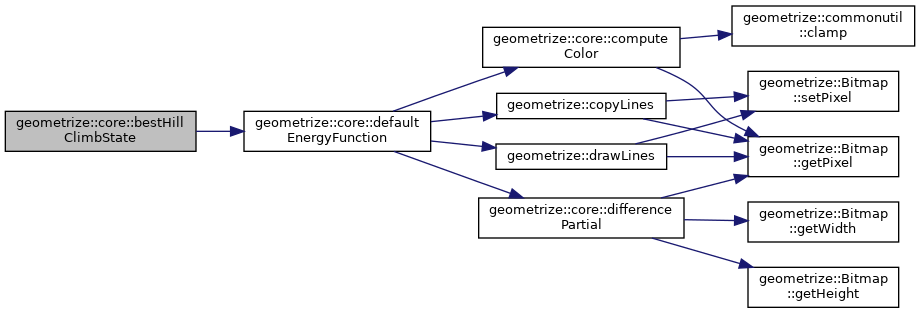Here is the caller graph for this function: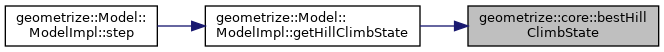geometrize::rgba geometrize::core::computeColor ( const geometrize::Bitmap & target, const geometrize::Bitmap & current, const std::vector< geometrize::Scanline > & lines, std::uint8_t alpha )

computeColor Calculates the color of the scanlines.

Parameters
 target The target image. current The current image. lines The scanlines. alpha The alpha of the scanline.
Returns
The color of the scanlines.
127 {
128  // Early out to avoid integer divide by 0
129  if(lines.empty()) {
130  return geometrize::rgba{0, 0, 0, 0};
131  }
132
133  std::int64_t totalRed{0};
134  std::int64_t totalGreen{0};
135  std::int64_t totalBlue{0};
136  std::int64_t count{0};
137  const std::int32_t a{static_cast<std::int32_t>(257.0f * 255.0f / static_cast<float>(alpha))};
138
139  // For each scanline
140  for(const geometrize::Scanline& line : lines) {
141  const std::int32_t y{line.y};
142  for(std::int32_t x = line.x1; x <= line.x2; x++) {
143  // Get the overlapping target and current colors
144  const geometrize::rgba t(target.getPixel(x, y));
145  const geometrize::rgba c(current.getPixel(x, y));
146
147  const std::int32_t tr{t.r};
148  const std::int32_t tg{t.g};
149  const std::int32_t tb{t.b};
150  const std::int32_t cr{c.r};
151  const std::int32_t cg{c.g};
152  const std::int32_t cb{c.b};
153
154  // Mix the red, green and blue components, blending by the given alpha value
155  totalRed += static_cast<std::int64_t>((tr - cr) * a + cr * 257);
156  totalGreen += static_cast<std::int64_t>((tg - cg) * a + cg * 257);
157  totalBlue += static_cast<std::int64_t>((tb - cb) * a + cb * 257);
158  count++;
159  }
160  }
161
162  const std::int32_t rr{static_cast<std::int32_t>(totalRed / count) >> 8};
163  const std::int32_t gg{static_cast<std::int32_t>(totalGreen / count) >> 8};
164  const std::int32_t bb{static_cast<std::int32_t>(totalBlue / count) >> 8};
165
166  // Scale totals down to 0-255 range and return average blended color
167  const std::uint8_t r{static_cast<std::uint8_t>(commonutil::clamp(rr, INT32_C(0), INT32_C(255)))};
168  const std::uint8_t g{static_cast<std::uint8_t>(commonutil::clamp(gg, INT32_C(0), INT32_C(255)))};
169  const std::uint8_t b{static_cast<std::uint8_t>(commonutil::clamp(bb, INT32_C(0), INT32_C(255)))};
170
171  return geometrize::rgba{r, g, b, alpha};
172 }
The Scanline class represents a scanline, a row of pixels running across a bitmap.
Definition: scanline.h:13
geometrize::rgba getPixel(std::uint32_t x, std::uint32_t y) const
getPixel Gets a pixel color value.
Definition: bitmap.cpp:40
T clamp(const T &value, const T &lower, const T &upper)
clamp Clamps a value within a range.
Definition: commonutil.h:41
std::uint8_t r
Definition: rgba.h:14
The rgba struct is a helper for manipulating RGBA8888 color data.
Definition: rgba.h:12

Here is the call graph for this function: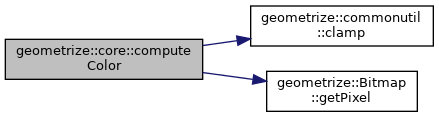Here is the caller graph for this function: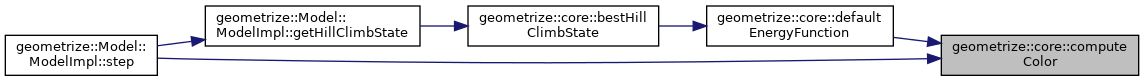double geometrize::core::defaultEnergyFunction ( const std::vector< geometrize::Scanline > & lines, const std::uint32_t alpha, const geometrize::Bitmap & target, const geometrize::Bitmap & current, geometrize::Bitmap & buffer, double score )

defaultEnergyFunction The default/built-in energy function that calculates a measure of the improvement adding the scanlines of a shape provides - lower energy is better.

Parameters
 lines The scanlines of the shape. alpha The alpha of the scanlines. target The target bitmap. current The current bitmap. buffer The buffer bitmap. score The score.
Returns
The energy measure.
115 {
116  const geometrize::rgba color(geometrize::core::computeColor(target, current, lines, alpha)); // Calculate best color for areas covered by the scanlines
117  geometrize::copyLines(buffer, current, lines); // Copy area covered by scanlines to buffer bitmap
118  geometrize::drawLines(buffer, color, lines); // Blend scanlines into the buffer using the color calculated earlier
119  return geometrize::core::differencePartial(target, current, buffer, score, lines); // Get error measure between areas of current and modified buffers covered by scanlines
120 }
void copyLines(geometrize::Bitmap &destination, const geometrize::Bitmap &source, const std::vector< geometrize::Scanline > &lines)
copyLines Copies source pixels to a destination defined by a set of scanlines.
Definition: rasterizer.cpp:112
void drawLines(geometrize::Bitmap &image, const geometrize::rgba color, const std::vector< geometrize::Scanline > &lines)
drawLines Draws scanlines onto an image.
Definition: rasterizer.cpp:74
geometrize::rgba computeColor(const geometrize::Bitmap &target, const geometrize::Bitmap &current, const std::vector< geometrize::Scanline > &lines, const std::uint8_t alpha)
computeColor Calculates the color of the scanlines.
Definition: core.cpp:122
The rgba struct is a helper for manipulating RGBA8888 color data.
Definition: rgba.h:12
double differencePartial(const geometrize::Bitmap &target, const geometrize::Bitmap &before, const geometrize::Bitmap &after, const double score, const std::vector< Scanline > &lines)
differencePartial Calculates the root-mean-square error between the parts of the two bitmaps within t...
Definition: core.cpp:198

Here is the call graph for this function: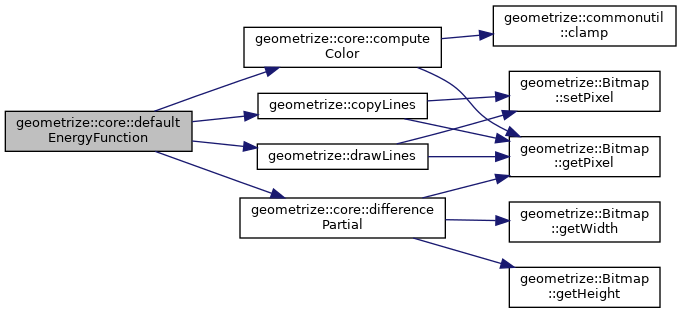Here is the caller graph for this function: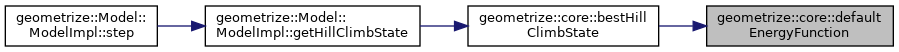double geometrize::core::differenceFull ( const geometrize::Bitmap & first, const geometrize::Bitmap & second )

differenceFull Calculates the root-mean-square error between two bitmaps.

Parameters
 first The first bitmap. second The second bitmap.
Returns
The difference/error measure between the two bitmaps.
175 {
176  assert(first.getWidth() == second.getWidth());
177  assert(first.getHeight() == second.getHeight());
178
179  const std::size_t width{first.getWidth()};
180  const std::size_t height{first.getHeight()};
181  std::uint64_t total{0};
182
183  for(std::size_t y = 0; y < height; y++) {
184  for(std::size_t x = 0; x < width; x++) {
185  const geometrize::rgba f(first.getPixel(x, y));
186  const geometrize::rgba s(second.getPixel(x, y));
187
188  const std::int32_t dr = {static_cast<std::int32_t>(f.r) - static_cast<std::int32_t>(s.r)};
189  const std::int32_t dg = {static_cast<std::int32_t>(f.g) - static_cast<std::int32_t>(s.g)};
190  const std::int32_t db = {static_cast<std::int32_t>(f.b) - static_cast<std::int32_t>(s.b)};
191  const std::int32_t da = {static_cast<std::int32_t>(f.a) - static_cast<std::int32_t>(s.a)};
192  total += (dr * dr + dg * dg + db * db + da * da);
193  }
194  }
195  return std::sqrt(static_cast<double>(total) / (static_cast<double>(width) * static_cast<double>(height) * 4.0)) / 255.0;
196 }
std::uint32_t getWidth() const
getWidth Gets the width of the bitmap.
Definition: bitmap.cpp:20
geometrize::rgba getPixel(std::uint32_t x, std::uint32_t y) const
getPixel Gets a pixel color value.
Definition: bitmap.cpp:40
The rgba struct is a helper for manipulating RGBA8888 color data.
Definition: rgba.h:12
std::uint32_t getHeight() const
getHeight Gets the height of the bitmap.
Definition: bitmap.cpp:25

Here is the call graph for this function: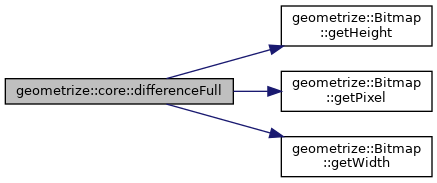Here is the caller graph for this function: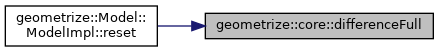double geometrize::core::differencePartial ( const geometrize::Bitmap & target, const geometrize::Bitmap & before, const geometrize::Bitmap & after, double score, const std::vector< Scanline > & lines )

differencePartial Calculates the root-mean-square error between the parts of the two bitmaps within the scanline mask. This is for optimization purposes, it lets us calculate new error values only for parts of the image we know have changed.

Parameters
 target The target bitmap. before The bitmap before the change. after The bitmap after the change. score The score. lines The scanlines.
Returns
The difference/error between the two bitmaps, masked by the scanlines.
204 {
205  const std::uint64_t rgbaCount{target.getWidth() * target.getHeight() * 4U};
206  std::uint64_t total{static_cast<std::uint64_t>((score * 255.0) * (score * 255.0) * rgbaCount)};
207  for(const geometrize::Scanline& line : lines) {
208  const std::int32_t y{line.y};
209  for(std::int32_t x = line.x1; x <= line.x2; x++) {
210  const geometrize::rgba t(target.getPixel(x, y));
211  const geometrize::rgba b(before.getPixel(x, y));
212  const geometrize::rgba a(after.getPixel(x, y));
213
214  const std::int32_t dtbr{static_cast<std::int32_t>(t.r) - static_cast<std::int32_t>(b.r)};
215  const std::int32_t dtbg{static_cast<std::int32_t>(t.g) - static_cast<std::int32_t>(b.g)};
216  const std::int32_t dtbb{static_cast<std::int32_t>(t.b) - static_cast<std::int32_t>(b.b)};
217  const std::int32_t dtba{static_cast<std::int32_t>(t.a) - static_cast<std::int32_t>(b.a)};
218
219  const std::int32_t dtar{static_cast<std::int32_t>(t.r) - static_cast<std::int32_t>(a.r)};
220  const std::int32_t dtag{static_cast<std::int32_t>(t.g) - static_cast<std::int32_t>(a.g)};
221  const std::int32_t dtab{static_cast<std::int32_t>(t.b) - static_cast<std::int32_t>(a.b)};
222  const std::int32_t dtaa{static_cast<std::int32_t>(t.a) - static_cast<std::int32_t>(a.a)};
223
224  total -= static_cast<std::uint64_t>(dtbr * dtbr + dtbg * dtbg + dtbb * dtbb + dtba * dtba);
225  total += static_cast<std::uint64_t>(dtar * dtar + dtag * dtag + dtab * dtab + dtaa * dtaa);
226  }
227  }
228
229  const double result{std::sqrt(static_cast<double>(total) / static_cast<double>(rgbaCount)) / 255.0};
230  return result;
231 }
std::uint32_t getWidth() const
getWidth Gets the width of the bitmap.
Definition: bitmap.cpp:20
The Scanline class represents a scanline, a row of pixels running across a bitmap.
Definition: scanline.h:13
geometrize::rgba getPixel(std::uint32_t x, std::uint32_t y) const
getPixel Gets a pixel color value.
Definition: bitmap.cpp:40
The rgba struct is a helper for manipulating RGBA8888 color data.
Definition: rgba.h:12
std::uint32_t getHeight() const
getHeight Gets the height of the bitmap.
Definition: bitmap.cpp:25

Here is the call graph for this function: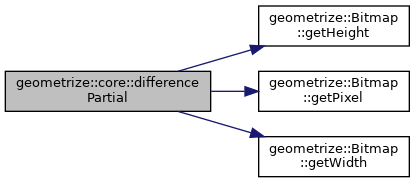Here is the caller graph for this function: